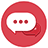# Office of International Relations

Known parameters:

1. Considering that the student's full-time duty is to be a student, the EU tried to calculate the hourly equivalent of 1 ECTS, arguing that if a student is considered a full-time worker he/she would have to work 40 hours.
2. Let’s calculate, based on the idea that a student at our university will work 45 hours a week to be successful in his courses.
3. He/she must have a total of 30 ECTS credits in a semester. (There may be differences)
4. A semester is 17 weeks at our university, excluding our Faculty of Medicine (15 weeks of lectures + 2 weeks of examaniations)
5. The total (average) time a student spends on a course to achieve the teaching outcomes of a course. This includes all the activities and pursuits of the student (taking classes, exams, exam preparation, midterms, homework, study, etc.)

Accordingly:

(45hours)x(17weeks)= 765 hours (time a student must spend to study in a semester)

1-ECTS= (765 hours /30 ECTS) = 25.5 hours

1-ECTS = 25.5 hours

The student spends this time on lectures, assignments, labs, exams, projects, and self-study. The teacher of each course lists the requirements of his own course and determines the ECTS credits on this basis.

For example: a 3-credit pool course; 10 assignments are given; There are 2 midterm exams (Saturday+Sunday) and let the student's self-study time be set as 30.

ECTS for the course:

(15 weeks of lessons)x(3 hours) = 45 hours / time spent by the student for the lesson

(10 assignments)x(3 hours) = 30 hours / time spent by the student making assignments

(2 midterm exams)x(3hours) = 6 hours / time spent for exams

= 30 hours of self-study at home, library, etc.

Total= 121 hours

1-ECTS= 25.5 hours

Canlı Görüşme Mesaj BırakınBize Sorun!* Gerekli
* Gerekli
* Gerekli
* Gerekli
* Opsiyonel